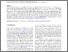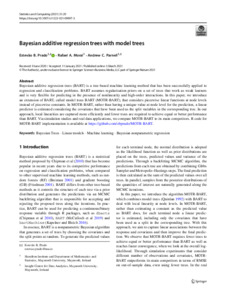# Bayesian additive regression trees with model trees

Prado, Estevão B. and de Andrade Moral, Rafael and Parnell, Andrew (2021) Bayesian additive regression trees with model trees. Statistics and Computing, 31 (3). ISSN 0960-3174Previewmore...Add this article to your Mendeley library

## Abstract

Bayesian additive regression trees (BART) is a tree-based machine learning method that has been successfully applied to regression and classification problems. BART assumes regularisation priors on a set of trees that work as weak learners and is very flexible for predicting in the presence of nonlinearity and high-order interactions. In this paper, we introduce an extension of BART, called model trees BART (MOTR-BART), that considers piecewise linear functions at node levels instead of piecewise constants. In MOTR-BART, rather than having a unique value at node level for the prediction, a linear predictor is estimated considering the covariates that have been used as the split variables in the corresponding tree. In our approach, local linearities are captured more efficiently and fewer trees are required to achieve equal or better performance than BART. Via simulation studies and real data applications, we compare MOTR-BART to its main competitors. R code for MOTR-BART implementation is available at https://github.com/ebprado/MOTR-BART.

Item Type: Article Bayesian Trees; Linear models; Machine learning; Bayesian nonparametric regression; Faculty of Science and Engineering > Mathematics and StatisticsFaculty of Science and Engineering > Research Institutes > Hamilton Institute 15498 https://doi.org/10.1007/s11222-021-09997-3 Andrew Parnell 15 Feb 2022 12:25 Statistics and Computing Springer Verlag YesItem control page